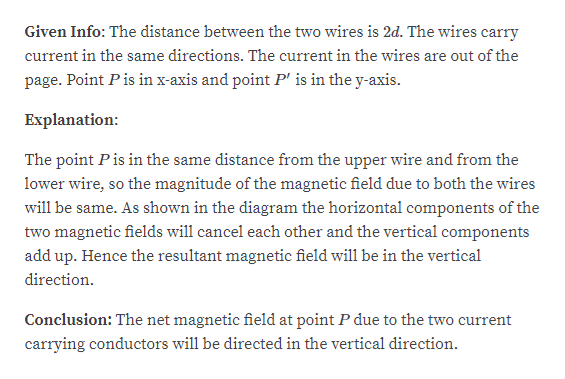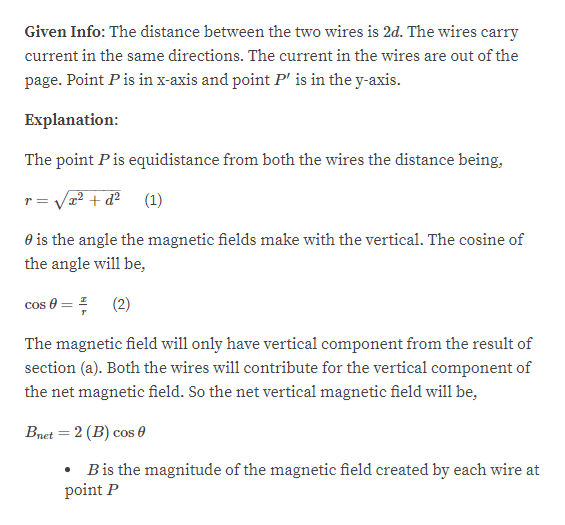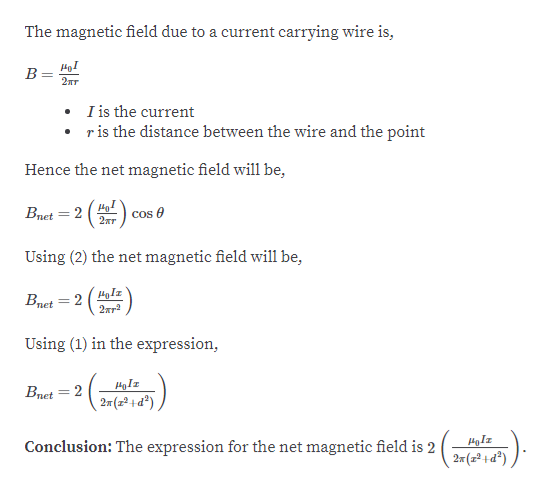Question
1 views

Two long, parallel wires
separated by a distance 2d carry
equal currents in the same direction.
An end view of the two wires
is shown in Figure P19.54, where
the currents are out of the page.
(a) What is the direction of the
magnetic field at P on the x - axis
set up by the two wires? (b) Find an
expression for the magnitude of the
field at P. (c) From your result to part (b), determine the field
at a point midway between the two wires. Does your result

check_circle

Step 1

Part A:help_outlineImage TranscriptioncloseGiven Info: The distance between the two wires is 2d. The wires carry current in the same directions. The current in the wires are out of the page. Point Pis in x-axis and point P' is in the y-axis. Explanation: The point Pis in the same distance from the upper wire and from the lower wire, so the magnitude of the magnetic field due to both the wires will be same. As shown in the diagram the horizontal components of the two magnetic fields will cancel each other and the vertical components add up. Hence the resultant magnetic field will be in the vertical direction. Conclusion: The net magnetic field at point P due to the two current carrying conductors will be directed in the vertical direction. fullscreen
Step 2

Part b:help_outlineImage TranscriptioncloseGiven Info: The distance between the two wires is 2d. The wires carry current in the same directions. The current in the wires are out of the page. Point Pis in x-axis and point P' is in the y-axis. Explanation: The point Pis equidistance from both the wires the distance being, (1) r = Va2 + d? O is the angle the magnetic fields make with the vertical. The cosine of the angle will be, cos 0 = = (2) The magnetic field will only have vertical component from the result of section (a). Both the wires will contribute for the vertical component of the net magnetic field. So the net vertical magnetic field will be, Bnet = 2 (B) cos 0 %3D Bis the magnitude of the magnetic field created by each wire at point P fullscreen
Step 3help_outlineImage TranscriptioncloseThe magnetic field due to a current carrying wire is, в 2лт • I is the current ris the distance between the wire and the point Hence the net magnetic field will be, (#). Bnet = 2 2тт cos e Using (2) the net magnetic field will be, HyIz Bnet = 22ar Using (1) in the expression, Bnet = 2 27(z² +d²) HgIz Conclusion: The expression for the net magnetic field is 2 27(z² +d²) fullscreen

### Want to see the full answer?

See Solution

#### Want to see this answer and more?

Solutions are written by subject experts who are available 24/7. Questions are typically answered within 1 hour.*

See Solution
*Response times may vary by subject and question.
Tagged in

### Physics